Anúncio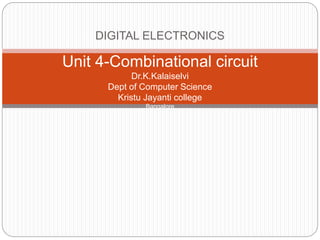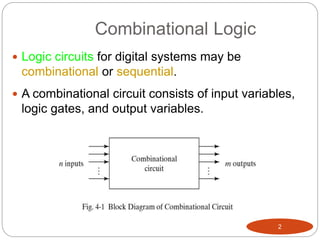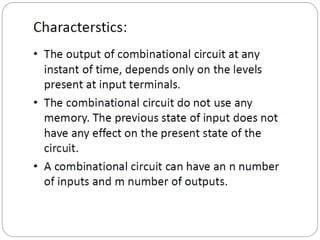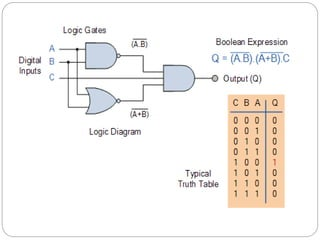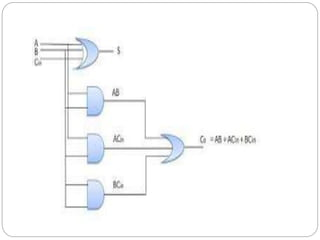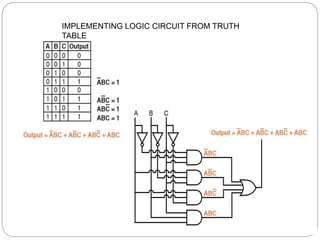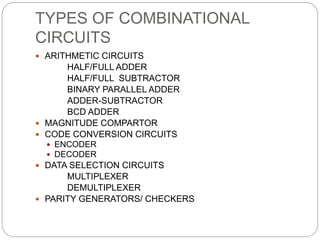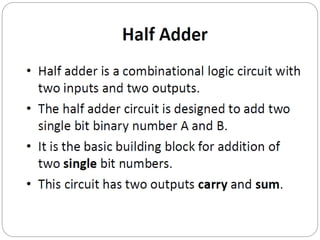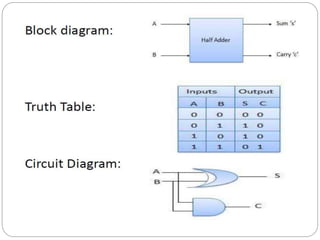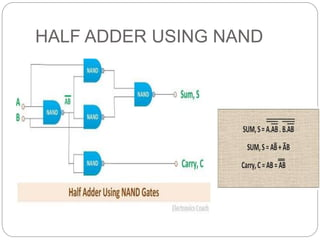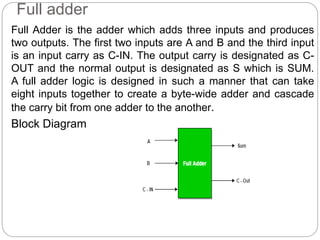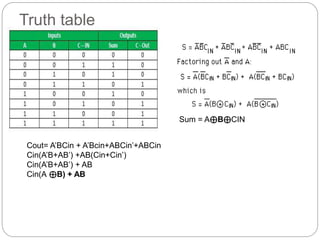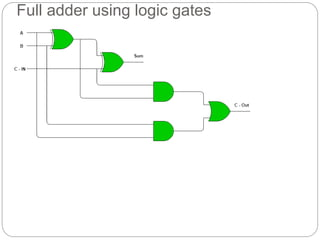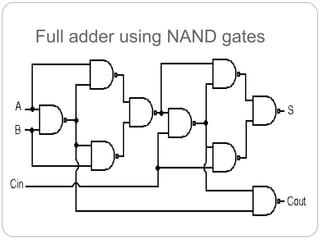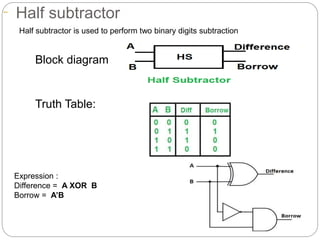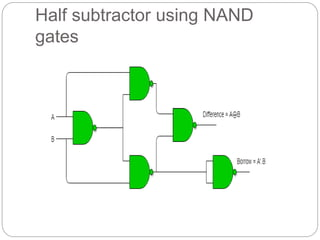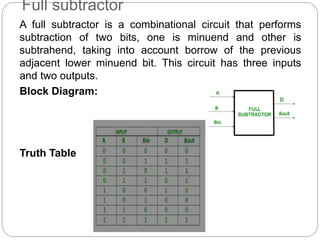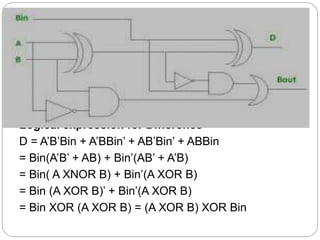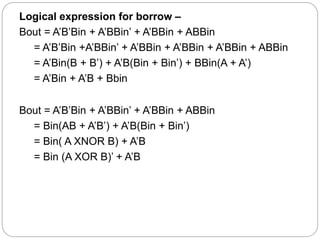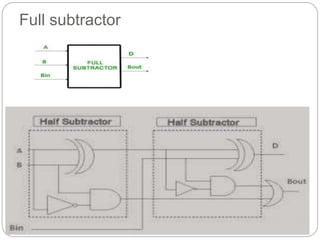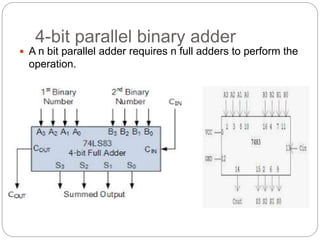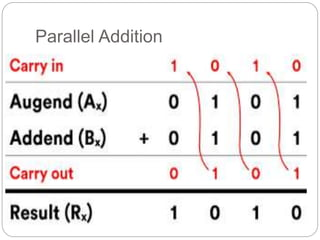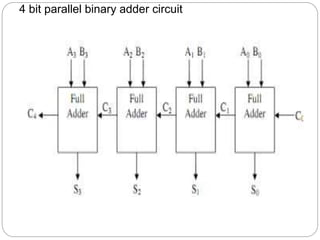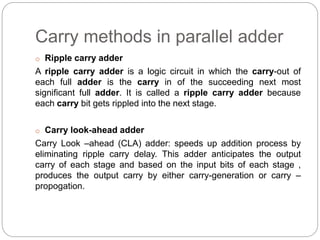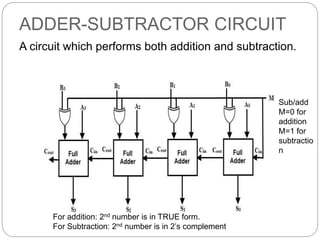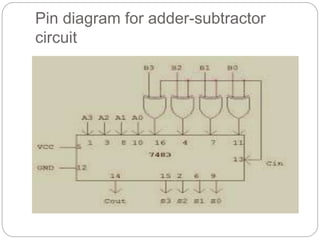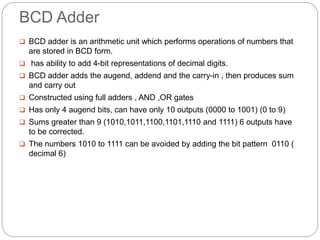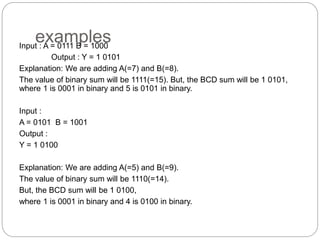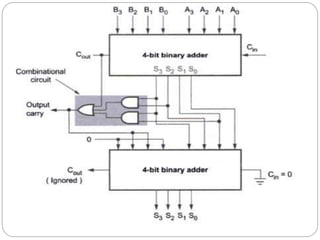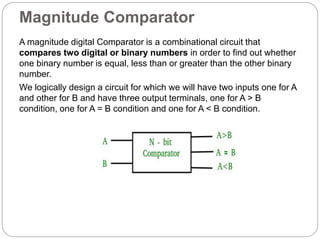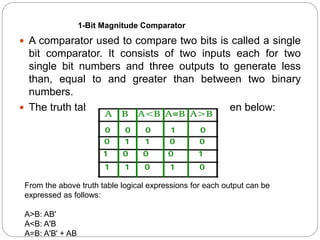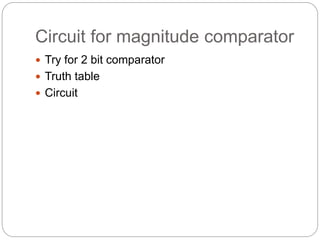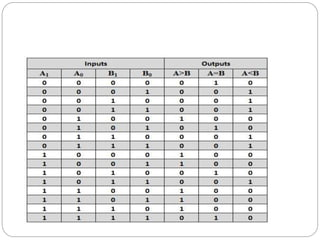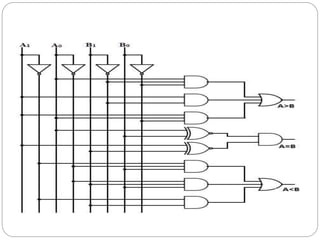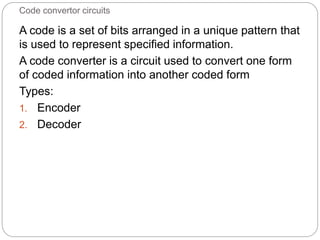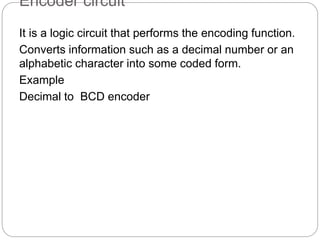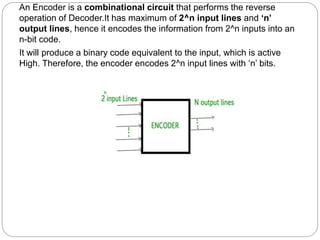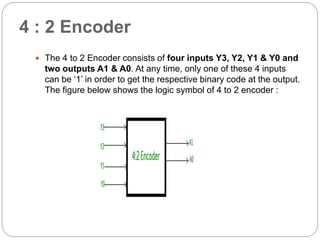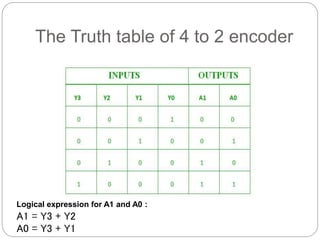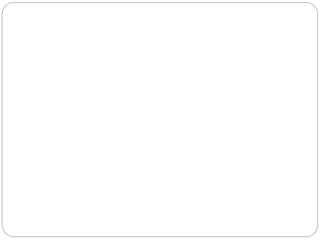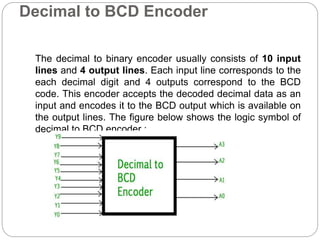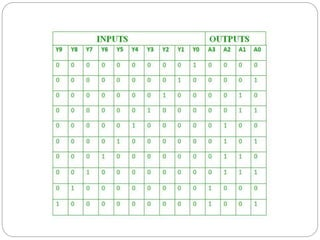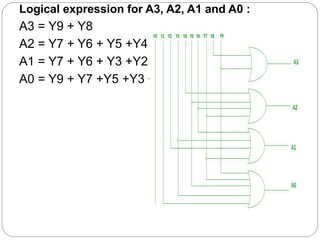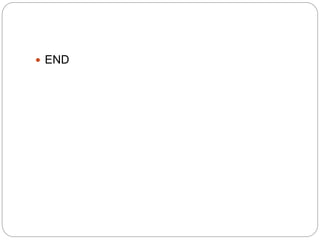1 de 44
Anúncio

### Unit 4 combinational circuit

1. DIGITAL ELECTRONICS Unit 4-Combinational circuit Dr.K.Kalaiselvi Dept of Computer Science Kristu Jayanti college Bangalore
2. Combinational Logic  Logic circuits for digital systems may be combinational or sequential.  A combinational circuit consists of input variables, logic gates, and output variables. 2
3. IMPLEMENTING LOGIC CIRCUIT FROM TRUTH TABLE
4. TYPES OF COMBINATIONAL CIRCUITS  ARITHMETIC CIRCUITS HALF/FULL ADDER HALF/FULL SUBTRACTOR BINARY PARALLEL ADDER ADDER-SUBTRACTOR BCD ADDER  MAGNITUDE COMPARTOR  CODE CONVERSION CIRCUITS  ENCODER  DECODER  DATA SELECTION CIRCUITS MULTIPLEXER DEMULTIPLEXER  PARITY GENERATORS/ CHECKERS
6. Full adder Full Adder is the adder which adds three inputs and produces two outputs. The first two inputs are A and B and the third input is an input carry as C-IN. The output carry is designated as C- OUT and the normal output is designated as S which is SUM. A full adder logic is designed in such a manner that can take eight inputs together to create a byte-wide adder and cascade the carry bit from one adder to the another. Block Diagram
7. Truth table Sum = A⊕B⊕CIN Cout= A’BCin + A’Bcin+ABCin’+ABCin Cin(A’B+AB’) +AB(Cin+Cin’) Cin(A’B+AB’) + AB Cin(A ⊕B) + AB
8. Full adder using logic gates
9. Full adder using NAND gates
10. Half subtractor Block diagram: Truth Table: Expression : Difference = A XOR B Borrow = A’B Half subtractor is used to perform two binary digits subtraction
11. Half subtractor using NAND gates
12. Full subtractor A full subtractor is a combinational circuit that performs subtraction of two bits, one is minuend and other is subtrahend, taking into account borrow of the previous adjacent lower minuend bit. This circuit has three inputs and two outputs. Block Diagram: Truth Table
13. Logical expression for difference – Logical expression for Difference- D = A’B’Bin + A’BBin’ + AB’Bin’ + ABBin = Bin(A’B’ + AB) + Bin’(AB’ + A’B) = Bin( A XNOR B) + Bin’(A XOR B) = Bin (A XOR B)’ + Bin’(A XOR B) = Bin XOR (A XOR B) = (A XOR B) XOR Bin
14. Logical expression for borrow – Bout = A’B’Bin + A’BBin’ + A’BBin + ABBin = A’B’Bin +A’BBin’ + A’BBin + A’BBin + A’BBin + ABBin = A’Bin(B + B’) + A’B(Bin + Bin’) + BBin(A + A’) = A’Bin + A’B + Bbin Bout = A’B’Bin + A’BBin’ + A’BBin + ABBin = Bin(AB + A’B’) + A’B(Bin + Bin’) = Bin( A XNOR B) + A’B = Bin (A XOR B)’ + A’B
15. Full subtractor
16. 4-bit parallel binary adder  A n bit parallel adder requires n full adders to perform the operation.
18. 4 bit parallel binary adder circuit
20. ADDER-SUBTRACTOR CIRCUIT A circuit which performs both addition and subtraction. Sub/add M=0 for addition M=1 for subtractio n For addition: 2nd number is in TRUE form. For Subtraction: 2nd number is in 2’s complement
21. Pin diagram for adder-subtractor circuit
22. BCD Adder  BCD adder is an arithmetic unit which performs operations of numbers that are stored in BCD form.  has ability to add 4-bit representations of decimal digits.  BCD adder adds the augend, addend and the carry-in , then produces sum and carry out  Constructed using full adders , AND ,OR gates  Has only 4 augend bits, can have only 10 outputs (0000 to 1001) (0 to 9)  Sums greater than 9 (1010,1011,1100,1101,1110 and 1111) 6 outputs have to be corrected.  The numbers 1010 to 1111 can be avoided by adding the bit pattern 0110 ( decimal 6)
23. examples Input : A = 0111 B = 1000 Output : Y = 1 0101 Explanation: We are adding A(=7) and B(=8). The value of binary sum will be 1111(=15). But, the BCD sum will be 1 0101, where 1 is 0001 in binary and 5 is 0101 in binary. Input : A = 0101 B = 1001 Output : Y = 1 0100 Explanation: We are adding A(=5) and B(=9). The value of binary sum will be 1110(=14). But, the BCD sum will be 1 0100, where 1 is 0001 in binary and 4 is 0100 in binary.
24. Magnitude Comparator A magnitude digital Comparator is a combinational circuit that compares two digital or binary numbers in order to find out whether one binary number is equal, less than or greater than the other binary number. We logically design a circuit for which we will have two inputs one for A and other for B and have three output terminals, one for A > B condition, one for A = B condition and one for A < B condition.
25.  A comparator used to compare two bits is called a single bit comparator. It consists of two inputs each for two single bit numbers and three outputs to generate less than, equal to and greater than between two binary numbers.  The truth table for a 1-bit comparator is given below: From the above truth table logical expressions for each output can be expressed as follows: A>B: AB' A<B: A'B A=B: A'B' + AB 1-Bit Magnitude Comparator
26. Circuit for magnitude comparator  Try for 2 bit comparator  Truth table  Circuit
27. Code convertor circuits A code is a set of bits arranged in a unique pattern that is used to represent specified information. A code converter is a circuit used to convert one form of coded information into another coded form Types: 1. Encoder 2. Decoder
28. Encoder circuit It is a logic circuit that performs the encoding function. Converts information such as a decimal number or an alphabetic character into some coded form. Example Decimal to BCD encoder
29. An Encoder is a combinational circuit that performs the reverse operation of Decoder.It has maximum of 2^n input lines and ‘n’ output lines, hence it encodes the information from 2^n inputs into an n-bit code. It will produce a binary code equivalent to the input, which is active High. Therefore, the encoder encodes 2^n input lines with ‘n’ bits.
30. 4 : 2 Encoder  The 4 to 2 Encoder consists of four inputs Y3, Y2, Y1 & Y0 and two outputs A1 & A0. At any time, only one of these 4 inputs can be ‘1’ in order to get the respective binary code at the output. The figure below shows the logic symbol of 4 to 2 encoder :
31. The Truth table of 4 to 2 encoder Logical expression for A1 and A0 : A1 = Y3 + Y2 A0 = Y3 + Y1
32. Decimal to BCD Encoder The decimal to binary encoder usually consists of 10 input lines and 4 output lines. Each input line corresponds to the each decimal digit and 4 outputs correspond to the BCD code. This encoder accepts the decoded decimal data as an input and encodes it to the BCD output which is available on the output lines. The figure below shows the logic symbol of decimal to BCD encoder :
33. Logical expression for A3, A2, A1 and A0 : A3 = Y9 + Y8 A2 = Y7 + Y6 + Y5 +Y4 A1 = Y7 + Y6 + Y3 +Y2 A0 = Y9 + Y7 +Y5 +Y3 + Y1
34.  END
Anúncio